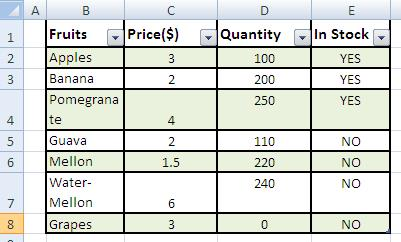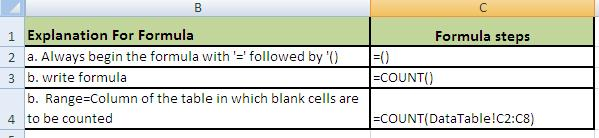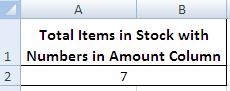# Count numbers excel cells

## Step by Step: How to Use Count in excel 2003, 2007 & 2010 for counting numeric or number cells

Count numbers excel cells: What is Count function? Excel Count, counts data rows where cells contain digit or numeric cells in the column on which this formula is applied. In other words Count is used to count cells in excel that have values and those values are numeric or have digits only. If you wish to count items available in store with blank cell instead of amounts it will count those items.

### Step 1 for counting Number cells in Excel:

(b) Formulate your tables: these may be on the same table or on a different worksheet or even different workbooks.
(c) For the sake of example we have created two Tables.
(d) Table from where data is to be retrieved:MS excel count number cells

(e) Table where data is to be fetched/desired.MS Excel count numeric data in cell

### Step 2 to Count Numeric Excel cells:

Ensure spellings for search values are the same in both the tables.

### Step 3 for doing calculation of numeric cells:

Make sure there is no extra space.

### Step 4 to count number cells in MS Excel:Count numbers in excel 2007 2010

### Final Formula: Let us have a look at the final formula in MS Excel to count number cellsMS Excel count only numbers

### Result:MS excel counting excel number cellsMS excel 2007 2010 counting number cells

### Advantages of using Count Numbers formula:

1. You may copy and paste Count formula anywhere within the same sheet or even workbook for counting numeric or digit values in any column of any worksheet.
2. A really swift and smooth process for finding and counting of blank entries across the sheets and workbooks with ease and perfection.